Courses

# Test: Structural Fasteners - 4

## 20 Questions MCQ Test Topicwise Question Bank for Civil Engineering | Test: Structural Fasteners - 4

Description
This mock test of Test: Structural Fasteners - 4 for Civil Engineering (CE) helps you for every Civil Engineering (CE) entrance exam. This contains 20 Multiple Choice Questions for Civil Engineering (CE) Test: Structural Fasteners - 4 (mcq) to study with solutions a complete question bank. The solved questions answers in this Test: Structural Fasteners - 4 quiz give you a good mix of easy questions and tough questions. Civil Engineering (CE) students definitely take this Test: Structural Fasteners - 4 exercise for a better result in the exam. You can find other Test: Structural Fasteners - 4 extra questions, long questions & short questions for Civil Engineering (CE) on EduRev as well by searching above.
QUESTION: 1

Solution:
QUESTION: 2

Solution:
QUESTION: 3

### Tacking rivets in tension members, are provided at a pitch in line not exceeding

Solution:
QUESTION: 4

The effective section of a fillet weld Is represented by a triangle ABC with sides S1, S2 and S3 such that S3 > S2 > S1.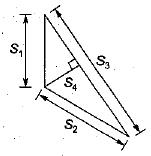If the allowable shear stress in weld material is τ, the resistance of weld per unit length is

Solution:
QUESTION: 5

The steel parts at right angles, are welded with fillet weld of 10 mm size. The throat thickness of the fillet weld should be

Solution:
QUESTION: 6

Which one of the following statements regarding the riveted joint shown in the given figure is correct?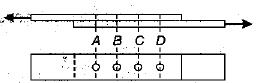Solution:

The rivets are assumed to resist the load equally in the elastic theory. Actually the outer rivets carry more load compared to rivets in the middle rows. When the load is increased, the more highly stressed rivets start yielding earlier and there is redistribution of load among rivets. The failure will occur when all rivets have yielded and the strength of the group of rivets is equal to the strength of one rivet multiplied by the number of rivets.

QUESTION: 7

Select the correct statement

Solution:
QUESTION: 8

When the bolts are subjected to reversal of stresses, the most suitable type of bolt is

Solution:
QUESTION: 9

A bolt is subjected to shear force V and tension T. The capacity of the bolt in resisting shear and tension respectively are V0 and T0. Which one of the following diagrams represents interaction relations?

Solution:

The interaction equation for a high tension bolt subjected to shear and tension is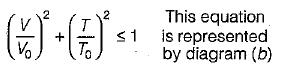According to IS : 800, the rivets and bolts subjected to both shear and tension shall be so proportioned that the calculated shear and axial forces do not exceed the respective allowable forces i.e.,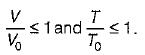Further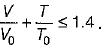So curve(d) represents the interaction equation.

QUESTION: 10

The actual thickness of butt weld as compared to the thickness of plate is usually

Solution:
QUESTION: 11

Bolts are most suitable to carry

Solution:
QUESTION: 12

Efficiency of a riveted joint, having the minimum pitch as per IS : 800, is

Solution:
QUESTION: 13

The ratio of yield stress in tension to compression in mild steel is

Solution:
QUESTION: 14

Consider the riveted joint shown in figure. The maximum permissible value of ‘p’ (rivet diameter 20 mm) is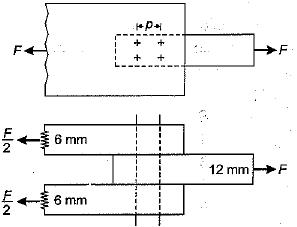Solution:
QUESTION: 15

If a bolt of nominal diameter 18 mm then gross dia for the standard clearance hole as per IS 800 : 2007 is

Solution: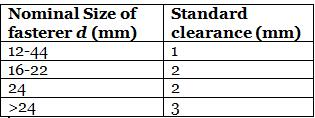QUESTION: 16

In which of the following cases is a structural fastener over designed?
1. When design is based on Unwin's formula
3. When reversal of stresses takes place
Select the correct answer using the codes given below,

Solution:
QUESTION: 17

20 mm diameter rivets are used to connect 10 mm thick piates. The permlssitcle stresses for rivets in shear and bearing are 80 MPa and 250 MPa respectively. The difference of rivet value in single shear and double shear is

Solution: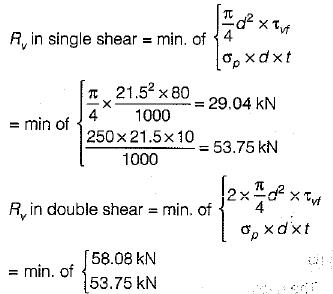Difference = 53.75 - 29.04 = 24.7 kN

QUESTION: 18

Which type of joint shows maximum efficiency in case of connection of two plates?

Solution:
QUESTION: 19

In which of the following case we avoid bolted connection

Solution:

Because bolted joints are susceptible to vibration and in case of frequent earthquake, it occurs.

QUESTION: 20

IS 800 : 2007 is based on

Solution:

New code of steel structures, IS : 800, has been formatted by considering limit state design method while earlier one was based on working stress method.